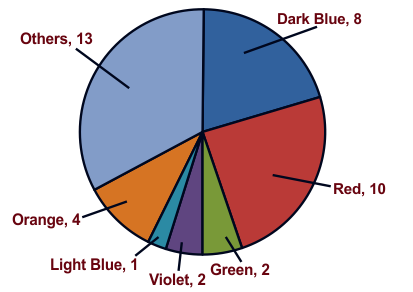# Humming Bird - HMO PDF Sample Papers for Class 6

Class 6 sample paper & practice questions for Humming Bird Mathematics Olympiad (HMO) level 1 are given below. Syllabus for level 1 is also mentioned for these exams. You can refer these sample paper & quiz for preparing for the exam.#### Resources:

##### Sample Questions from Olympiad Success:
 Q.1 Q.2 Q.3 Q.4 Q.5 Q.6 Q.7 Q.8 Q.9 Q.10
 Q.1 Ram was playing with 2 dice. He threw it 10 times and following were the results (3, 5), (1, 6), (2, 2), (3, 6), (6, 3), (6, 1), (2, 5), (1, 3), (2, 1), (6, 6) Which number appears the most number of times? a) 1 b) 2 c) 3 d) 6
 Q.2 Which property is reflected in the following? 7 x 5 = 5 x 7 a) Closure b) Associative c) Distributive d) Commutative
 Q.3 Form the equations: Sum of 2 times x and 3 times y is 1. a) 2x = 3y + 1 b) 3x + 2y = 1 c) 2x + 3y = 1 d) 3x = 2y + 1
 Q.4 The sum of first 5 prime number is: a) 26 b) 28 c) 11 d) 18
 Q.5 Find the ratio of 500 mL to 2 L a) 4 : 3 b) 3 : 4 c) 4 : 1 d) 1 : 4
 Q.6 What is the value of 81265 x 169 - 81265 x 69? a) 8126500 b) 81265000 c) Zero d) 100
 Q.7 Which of these are favourites of equal number of kids?a) Red and Orange b) Red and Dark Blue c) Violet and Light Blue d) Violet and Green
 Q.8 If a bicycle wheel has 48 spokes, then the angle between a pair of two consecutive spokes is: a) 11/2 b) 15/2 c) 2/11 d) 2/15
 Q.9 The cost of 105 envelopes is Rs. 35. How many envelopes can be purchased for Rs.10? a) 12 b) 40 c) 50 d) 30
 Q.10 The lines which do not intersect and have equal distance between them are called: a) perpendicular lines b) parallel lines c) intersecting lines d) straight linesSample PDF of Humming Bird - Humming Bird Mathematics Olympiad (HMO) PDF Sample Papers for Class 6:

 Q.1 )d Q.2 )d Q.3 )c Q.4 )d Q.5 )d Q.6 )a Q.7 )d Q.8 )b Q.9 )d Q.10 )b

Q.1 : d | Q.2 : d | Q.3 : c | Q.4 : d | Q.5 : d | Q.6 : a | Q.7 : d | Q.8 : b | Q.9 : d | Q.10 : b